Practice the AP 7th Class Maths Bits with Answers 12th Lesson Symmetry on a regular basis so that you can attempt exams with utmost confidence.

## AP Board 7th Class Maths Bits 12th Lesson Symmetry

Multiple Choice Questions :

Question 1.
The line which cuts the figures exactly into two identicals is called _________
(A) line of symmetry
(B) axis of symmetry
(C) rotational symmetry
(D) A or B
(D) A or B

Question 2.
Number of lines of symmetry of-an equilateral triangle is _________
(A) 3
(B) 4
(C) 5
(D) 6
(A) 3

Question 3.
Number of lines of symmetry of a square is _________
(A) 3
(B) 4
(C) 5
(D) 6
(B) 4

Question 4.
If we rotate a figure, about a fixed point by a certain angle and the figure looks exactly the same as before, we say that the figure has _________ symmetry.
(A) line of symmetry
(B) axis of symmetry
(C) rotational symmetry
(D) none
(C) rotational symmetry

Question 5.
The order of rotational symmetry of an equilateral triangle is _________
(A) 1
(B) 2
(C) 4
(D) 3
(D) 3

Question 6.
Angle of rotational symmetry of an equilateral triangle is _________
(A) 30°
(B) 60°
(C) 90°
(D) 120°
(D) 120°Question 7.
The order of rotational symmetry of a square is _________
(A) 4
(B) 3
(C) 2
(D) 1
(A) 4

Question 8.
Angle of rotational symmetry of a square is _________
(A) 30°
(B) 60°
(C) 90°
(D) 120°
(C) 90°

Question 9.
Which of the following is an example for the figures which have point of symmetry ?
(A) rectangle
(B) square
(C) regular hexagon
(D) all of the above
(D) all of the above

Question 10.
Examples for the figures which have line and point of symmetry _________
(A) square
(B) equilateral triangle
(C) circles
(D) all of the above
(D) all of the above

Question 11.
Number of axes of symmetry for a circle _________
(A) 1
(B)2
(C) 0
(D) infinite
(D) infinite

Question 12.
Which of the following figures has symmetry ?
(A) Match box
(B) Cricket ball
(C) A wheel
(D) All of the above
(D) All of the above

Question 13.
Number of axes of symmetry of the letter ‘M’ is ___________
(A) 0
(B) 2
(C) 1
(D) 3
(C) 1

Question 14.
WhIch of the following has a line of symmetry?
(A) P
(B) F
(C) M
(D) Q
(C) M

Question 15.
Which letter has no line of symmetry?
(A) A
(B) B
(C) C
(D) F
(D) F

Question 16.
Which of the following figure has axes of symmetry?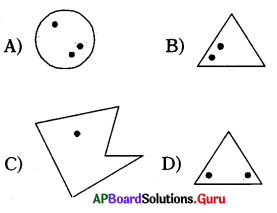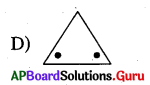Question 17.
What is the angle of a rotational symmetry of a parallelogram?
(A) 180°
(B) 90°
(C) 45°
(D) 120°
(A) 180°Question 18.
Which of the following has rotational symmetry?
(A) Wheel
(B) Square
(C) Circle
(D) All the above
(D) All the above

Question 19.
Order of rotational symmetry of ‘X’ is _________
(A) 4
(B) 2
(C) 3
(D) 6
(A) 4

Question 20.
Which of the following statement Is wrong?
(A) Every square has an axes of symmetry
(B) The letter ‘Z’ has no line of symmetry
(C) the letter ‘E’ has no rotational symmetry
(D) Every figure has an axes of symmetry
(D) Every figure has an axes of symmetry

Question 21.
Number of axes of symmetry of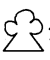(A) 1
(B) 2
(C) 3
(D) 4
(A) 1

Question 22.
Number of axes of symmetry of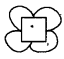(A) 1
(B) 2
(C) 3
(D) 4
(D) 4

Question 23.
Number of axes. of symmetry of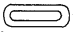(A) 1
(B) 2
(C) 3
(D) 4
(B) 2

Question 24.
Number of axes of smmetry of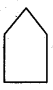(A) 2
(B) 3
(C) 4
(D) 5
(D) 5Question 25.
Number of axes of symmetry of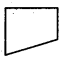(A) 1
(B) 0
(C) 2
(D) 3
(B) 0

Question 26.
Which of the following has a line of symmetry?
(A) P
(B) F
(C) M
(D) Q
(C) M

Question 27.
Dotted line given Is the line of symmetry, the other dot lies in is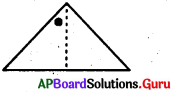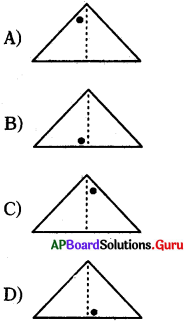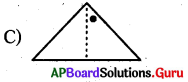Question 28.
Which of the following alphabet has no line of symmetry?
(A) A
(B) B
(C) C
(D) D
(D) D

Question 29.
Which of the following figure satisfies the property rotational symmetry ?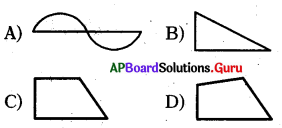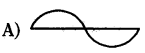Question 30.
How many axes of symmetry does the following regular pentagon have ?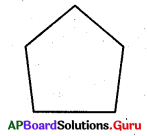(A) 2
(B) 3
(C) 4
(D) 5
(D) 5

Reasoning Questions:

Question 1.
if actual time in clock is 7 hours 30 minutes, then what is the time shown in mirror?
(A) 4hrs 30min
(B) 5 hrs 30 min
(C) 6hrs 30min
(D) 3hrs 30min
(A) 4hrs 30min

Question 2.
If actual time in clock is 5 hours 25 minutes, then what is the time shown in mirror?
(A) 5 hrs 35 mm
(B) 6 hrs 35 min
(C) 4 hrs 35 min
(D) 3 hrs 35 min
(B) 6 hrs 35 min

Question 3.
If actual time in clock is 12 hours 20 minutes, then what is the time shown in mirror?
(A) 12 hrs 40 min
(B) 10 hrs 40 min
(C) 11 hrs 40 min
(D) 1 hr 40 min
(C) 11 hrs 40 min

Question 4.
If actual time in clock is 4 hours 40 minutes, then what is the time shown in mirror?
(A) 7 hrs 20 min
(B) 6 hrs 20 min
(C) 5 hrs 40 min
(D) 7 hrs 40 min
(A) 7 hrs 20 min

Question 5.
If actual time in clock is 10 hours 26 minutes, then what is the time shown in mirror?
(A) 3 hrs 26 min
(B) 3 hrs 34 min
(C) 1 hr 26 min
(D) 1 hr 34 min
(D) 1 hr 34 min

Question 6.
If the time shown in mirror is 2 hours 35 minutes, then what is the actual time in clock?
(A) 9 hrs 25 min
(B) 9 hrs 35 min
(C) 8 hrs 35 min
(D) 8 hrs 25 min
(A) 9 hrs 25 minQuestion 7.
If the time shown in mirror is 8 hours 32 minutes, then what is the actual time in clock?
(A) 4 hrs 28 min
(B) 3 hrs 28 min
(C) 2 hrs 28 min
(D) 5 hrs 28 min
(B) 3 hrs 28 min

Question 8.
If the time shown in mirror is 11 hours 44 minutes, then what is the actual time in clock?
(A) 10 hrs 16 min
(B) 11 hrs 16 min
(C) 12 hrs 16 min
(D) 1 hr 16 min
(C) 12 hrs 16 min

Question 9.
If the time shown in mirror is 9 hours 16 minutes, then what is the actual time in clock?
(A) 5 hrs 16 min
(B) 4 hrs 16 min
(C) 3 hrs 44 min
(D) 2 hrs 44 min
(D) 2 hrs 44 min

Question 10.
If the time shown in mirror is 3 hours 29 minutes, then what is the actual time in clock?
(A) 8 hrs 31 min
(B) 9 hrs 31 min
(C) 10 hrs 31 min
(D) 7 hrs 31 min
(A) 8 hrs 31 min

Fill in the blanks :

Question 1.
A polygon with all equal sides and equal angles is called _________
Regular polygon

Question 2.
Number of lines of symmetry of a regular pentagon is _________
5

Question 3.
Number of lines of symmetry of a regular hexagon is _________
6

Question 4.
If a line divides the given figure into two coincidental parts, then the figure is said to be _________
symmetrical figure

Question 5.
The angle of turning during rotation is called _________
The angle of rotationQuestion 6.
The order of rotational symmetry of a rectangle is _________
2

Question 7.
The angle of rotational symmetry of a rectangle is _________
180°

Question 8.
The order of rotational symmetry of a Regular Hexagon is _________
6

Question 9.
The angle of rotational symmetry of regular hexagon is _________
60°

Question 10.
If any figure having 2nd order rotational symmetry, then it must have _________
Point of symmetry

Question 11.
Patterns are formed by arranging congruent figures side by side in all the directions to spread upon an area without any overlaps or gaps is called _________
Tessellation

Question 12.
Order of rotational symmetry of X is _________
4

Question 13.
Number of lines of symmetry for H is _________
2

Question 14.
Number of axes of symmetry of _________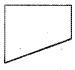0

Question 15.
The order of rotational symmetry = _________
$$\frac{360^{\circ}}{\text { Angle of rotational symmetry }}$$

Match the following :

Question 1.
Number of lines of symmetry of _________

 1. Equilateral triangle (A) 0 2. Square (B) 1 3. Rectangle (C) 2 4. Regular Pentagon (D) 3 5. Regular Hexagon (E) 4 6. Scalene triangle (F) 5 7. Isosceles triangle (G) 6